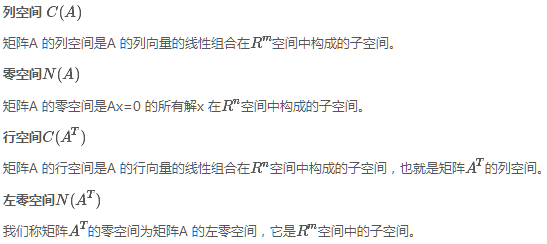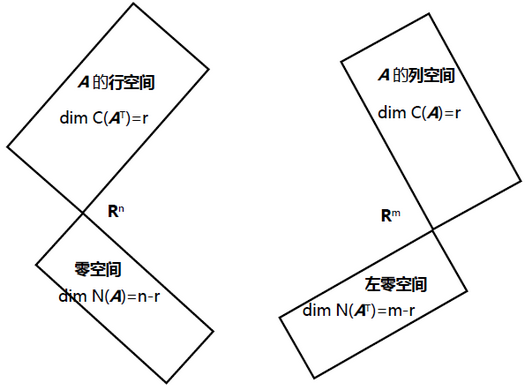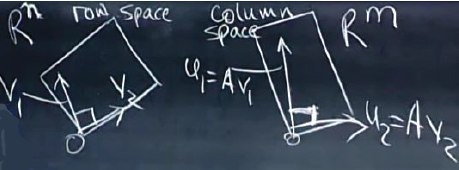13 篇文章 4 订阅

# 第二十八讲：正定矩阵和最小值

## 1.正定矩阵

### 1.1正定性的判断

$\color{red}{正定矩阵判定方法}$

• 1）特征值方法： λi>0 $λ_i>0$

• 3）主元方法：矩阵消元后主元均大于零；

• 4）新方法： xTAx>0 $x^TAx>0$ x $x$ 是任意向量，除零向量外。
大多数情况下使用4)来定义正定性，而用前三条来验证正定性。

1.矩阵的所有特征值大于零则矩阵正定： λ1>0,λ2>0 $\lambda_1>0,\lambda_2>0$
2.矩阵的所有顺序主子阵（leading principal submatrix）的行列式（即顺序主子式，leading principal minor）大于零则矩阵正定： a>0, acb2>0 $a>0,\ ac-b^2>0$
3.矩阵消元后主元均大于零： a>0, acb2a>0 $a>0,\ \frac{ac-b^2}{a}>0$
4. xTAx>0 $x^TAx>0$

### 1.2 最小值的判定及其几何意义

e=a2+b2 $e=\sqrt{a^2+b^2}$，a是长轴的长度，b是短轴的长度。

• 1）来试试18$18$，此时矩阵为 A= $A=\begin{bmatrix}2& 6\\6& 18\end{bmatrix}$ detA=0 $\det A=0$，此时的矩阵成为半正定矩阵（positive semi-definite）。矩阵奇异，其中一个特征值必为 0 $0$，从迹得知另一个特征值为20$20$。矩阵的主元只有一个，为 2 $2$
计算xTAx$x^TAx$，得

[x1x2][x1x2]=2x21+12x1x2+18x22

这样我们得到了一个关于 x1,x2 $x_1,x_2$的函数 f(x1,x2)=2x21+12x1x2+18x22 $f(x_1,x_2)=2x_1^2+12x_1x_2+18x_2^2$，这个函数不再是线性的，在本例中这是一个纯二次型（quadratic）函数，它没有线性部分、一次部分或更高次部分（ Ax $Ax$是线性的，但引入 xT $x^T$后就成为了二次型）。
当?取18时，判定1、2、3都是 $\color{red}{“刚好不及格”}$

00 $\color{red}{半正定矩阵：不是正定矩阵，是对称的，是成为正定矩阵的临界点，奇异矩阵，有一个特征值为0，其他特征 值大于等于0。}$

• 2)我们可以先看“ $\color{red}{一定不及格}$”的样子，令 ?=7 $?=7$，矩阵为 A= $A=\begin{bmatrix}2& 6\\6& 7\end{bmatrix}$，二阶顺序主子式变为 22 $-22$，显然矩阵不是正定的，此时的函数为 f(x1,x2)=2x21+12x1x2+7x22 $f(x_1,x_2)=2x_1^2+12x_1x_2+7x_2^2$，如果取 x1=1,x2=1 $x_1=1,x_2=-1$则有 f(1,1)=212+7<0 $f(1,-1)=2-12+7<0$

• 3)再来看一下“ $\color{red}{一定及格}$”的情形，令 ?=20 $?=20$，矩阵为 A= $A=\begin{bmatrix}2& 6\\6& 20\end{bmatrix}$，行列式为 detA=4 $\det A=4$，迹为 trace(A)=22 $trace(A)=22$，特征向量均大于零，矩阵可以通过测试。此时的函数为 f(x1,x2)=2x21+12x1x2+20x22 $f(x_1,x_2)=2x_1^2+12x_1x_2+20x_2^2$，函数在除 (0,0) $(0,0)$外处处为正。

• 一阶导数为零且二阶导数为正 dudx=0,d2udx2>0 $\frac{\mathrm{d}u}{\mathrm{d}x}=0, \frac{\mathrm{d}^2u}{\mathrm{d}x^2}>0$

• 在线性代数中我们遇到了了多元函数 f(x1,x2,,xn) $f(x_1,x_2,\cdots,x_n)$，要取极小值需要二阶偏导数矩阵为正定矩阵

• 一阶偏导为0；

• 对于二阶导数，这个矩阵型为[fxxfyxfxyfyy]$\begin{bmatrix}f_{xx}& f_{xy}\\f_{yx}&f_{yy}\end{bmatrix}$，显然，矩阵中的主对角线元素（纯二阶导数）必须为正，并且主对角线元素必须足够大来抵消混合导数的影响。同时还可以看出，因为二阶导数的求导次序并不影响结果，所以矩阵必须是对称的。

以此类推，现在我们就可以计算 n×n $n\times n$阶矩阵了。

### 1.3 正定矩阵的拓展

• 先来计算矩阵的顺序主子式，分别为 2,3,4 $2,3,4$；再来计算主元，分别为 2,32,43 $2,\frac{3}{2},\frac{4}{3}$；计算特征值， λ1=22,λ2=2,λ3=2+2 $\lambda_1=2-\sqrt 2,\lambda_2=2,\lambda_3=2+\sqrt 2$。(正定)

• 计算 xTAx=2x21+2x22+2x232x1x22x2x3 $x^TAx=2x_1^2+2x_2^2+2x_3^2-2x_1x_2-2x_2x_3$

• 图像是四维的抛物面，当我们在 f(x1,x2,x3)=1 $f(x_1,x_2,x_3)=1$处截取该面，将得到一个椭圆体。得到的图形则是一个扁的橄榄球，有一个长轴，另外两个轴相等，类似于一个矩阵有一重复的特征值，另一个不同（3 个特征值）。如果是球的话，那就是单位矩阵，所有的特征值相同。

$\color{red}{一般的情况下，三个特征值都不相同，它相当于有一个长轴，一个中轴，一个短轴，三个轴的方向就是特征向量的方向，轴的长度由特征值大小来决定。}$

A=QΛQT $A=Q\Lambda Q^T$是特征值相关章节中最重要的公式。

## 2. 本章总结

1. $\color{red}{正定矩阵判定方法}$

• 1）特征值方法： λi>0 $λ_i>0$

• 3）主元方法：矩阵消元后主元均大于零；

• 4）新方法： xTAx>0 $x^TAx>0$ x $x$ 是任意向量，除零向量外。
大多数情况下使用4)来定义正定性，而用前三条来验证正定性。

2. 最小值的判定：一阶偏导为0；二阶偏导大于0。

3. 主轴定理：
将矩阵A$A$(对称矩阵)分解为 A=QΛQT $A=Q\Lambda Q^T$，可以发现上面说到的各种元素都可以表示在这个分解的矩阵中，我们称之为 $\color{red}{主轴定理}$（principal axis theorem），即特征向量说明主轴的方向、特征值说明主轴的长度

# 第二十九讲：相似矩阵和若尔当形

• 我们将正定矩阵分解为 A=SΛS1 $A=S\Lambda S^{-1}$，引入其逆矩阵 A1=SΛ1S1 $A^{-1}=S\Lambda^{-1}S^{-1}$，我们知道正定矩阵的特征值均为正值，所以其逆矩阵的特征值也必为正值（即原矩阵特征值的倒数）所以，正定矩阵的逆矩阵也是正定的。

• 如果 A, B $A,\ B$均为正定矩阵，那么 A+B $A+B$呢？
我们可以从判定 xT(A+B)x $x^T(A+B)x$入手，根据条件有 xTAx>0, xTBx>0 $x^TAx>0,\ x^TBx>0$，将两式相加即得到 xT(A+B)x>0 $x^T(A+B)x>0$。所以正定矩阵之和也是正定矩阵。

• 再来看有 m×n $m\times n$矩阵 A $A$，则ATA$A^TA$(最早出现在最小二乘法)具有什么性质？
我们在投影部分经常使用 ATA $A^TA$，这个运算会得到一个对称矩阵，这个形式的运算用数字打比方就像是一个平方，用向量打比方就像是向量的长度平方，而对于矩阵，有 ATA $A^TA$正定：在式子两边分别乘向量及其转置得到 xTATAx $x^TA^TAx$，分组得到 (Ax)T(Ax) $(Ax)^T(Ax)$，相当于得到了向量 Ax $Ax$的长度平方，则 |Ax|20 $|Ax|^2\geq0$。要保证模不为零，则需要 Ax $Ax$的零空间中仅有零向量，即 A $A$的各列线性无关（rank(A)=n$rank(A)=n$）即可保证 |Ax|2>0 $|Ax|^2>0$ ATA $A^TA$正定。

• 另外，在矩阵数值计算中，正定矩阵消元不需要进行“行交换”操作，也不必担心主元过小或为零，正定矩阵具有良好的计算性质。

## 1. 相似矩阵

1.对于在对角化一讲（第二十二讲）中学过的式子 S1AS=Λ $S^{-1}AS=\Lambda$ S $S$是特征向量组成的矩阵），则有A$A$相似于 Λ $\Lambda$

### 1.1两大性质

1 $\color{red}{性质：1）相似矩阵具有相同的特征值；（注意特征向量并不相同）}$

2B=M1AMBMA $\color{red}{性质：2）B=M^{-1}AM， B 的特征向量等于M 的逆乘以矩阵A 的特征向量；}$

### 1.2当矩阵A有重复的特征值

• 第一族仅有一个矩阵  $\begin{bmatrix}4& 0\\0& 4\end{bmatrix}$，它只与自己相似（因为 M1M=4M1IM=4I= $M^{-1}\begin{bmatrix}4& 0\\0& 4\end{bmatrix}M=4M^{-1}IM=4I=\begin{bmatrix}4& 0\\0& 4\end{bmatrix}$，所以无论 M $M$如何取值该对角矩阵都只与自己相似）；

• 另一族就是剩下的诸如$\begin{bmatrix}4& 1\\0& 4\end{bmatrix}$的矩阵，它们都是相似的。在这个“大家族”中，  $\begin{bmatrix}4& 1\\0& 4\end{bmatrix}$是“最好”的一个矩阵(右上角为1)，称为 $\color{red}{若尔当形}$

• 继续上面的例子，我们在在出几个这一族的矩阵（若尔当认为它们并不是相似的，因为若尔当块大小不一样） , ,  $\begin{bmatrix}4& 1\\0& 4\end{bmatrix},\ \begin{bmatrix}5& 1\\-1& 3\end{bmatrix},\ \begin{bmatrix}4& 0\\17& 4\end{bmatrix}$，我们总是可以构造出一个满足 trace(A)=8, detA=16 $trace(A)=8,\ \det A=16$的矩阵，这个矩阵总是在这一个“家族”中。

## 2.若尔当形

• $\color{red}{若尔当块}$的定义型为:它只有一个重复的特征值，对角线上全是 λi $λ_i$，下面是0，上面是1，它的对角线上都是同一个数，只有一个特征向量。

Ji=λi1λi1λiλi

它的对角线上只为同一个数，仅有一个特征向量。

• J $\color{red}{若尔当阵J}$：由若尔当块构成的矩阵，特征值位于对角线上，对角线上方有若干个1，若尔当块的数量等于特征向量的个数，因为每一块对应于一个特征向量。

J=J1J2Jd

• $\color{red}{若尔当定理}$：每个方阵 A $A$ 都相似于一个若尔当阵J$J$。如果方阵 A $A$n$n$ 个互不相同的特征值，那么它是一个可对角化的矩阵，对应的若尔当阵就是对角阵 ΛJ=Λd=n $Λ，J=Λ，d=n$。(若尔当块的个数即为矩阵特征值的个数。)

$\color{red}{若尔当研究了所有情况，包含特征值重复的情况，此时特征向量的个数变少，这就是若尔当的理论。}$

## 3.本章总结

1. 正定矩阵的性质（a) A, B $A,\ B$均为正定矩阵，那么 A+B $A+B$ A1 $A^{-1}$的情况；b)矩阵 A $A$m×n$m\times n$，秩为n，则 ATA $A^TA$是否正定）；
2. 相似矩阵的2个性质和分类；
3. 若尔当阵，若尔当矩阵，若尔当定理。

# 第三十讲：奇异值分解

## 1. A=UΣVT $A=U\varSigma V^T$的推导

• 在正定一讲中（第二十八讲）我们知道一个正定矩阵可以分解为 A=QΛQT $A=Q\Lambda Q^T$的形式，由于 A $A$对称性其特征向量是正交的，且其Λ$\Lambda$矩阵中的元素皆为正，这就是正定矩阵的奇异值分解。在这种特殊的分解中，我们只需要一个正交矩阵 Q $Q$就可以使等式成立。

• 在对角化一讲中（第二十二讲），我们知道可对角化的矩阵能够分解为A=SΛST$A=S\Lambda S^T$的形式，其中 S $S$的列向量由A$A$的特征向量组成，但 S $S$并不是正交矩阵，所以这不是我们希望得到的奇异值分解。• 1) A $A$m×n$m×n$ 的矩阵，在行空间中找个典型变量，记为 v1 $v_1$，然后变换到列空间的某向量，记为 u1 $u_1$，有 u1=Av1 $u_1=Av_1$Av $Av$变换过程中，我希望转换得到的正交单位向量，所以 u1,u2.. $u_1,u_2..$是单位正交基，同时 v1,v2.. $v_1,v_2..$也是单位正交基， Av1 $Av_1$ 等于 u1 $u_1$ 的一个倍数（可以理解为：在奇异值分解中，要找的是行空间的一组正交基，然后变换成列空间的一组正交基。现在要做的是，在 A $A$的列空间中找到一组特殊的正交基v1,v2,,vr$v_1,v_2,\cdots,v_r$，这组基在 A $A$的作用下可以转换为A$A$的行空间中的一组正交基 u1,u2,,ur $u_1,u_2,\cdots,u_r$）。这种转换关系写成矩阵形式就是：

A[v1 v2  vr]=[σ1u1 σ2u2  σrur]=[u1 u2  ur]σ1σ2σr(1)

Av1=σ1u1, Av2=σ2u2,,Avr=σrur $Av_1=\sigma_1u_1,\ Av_2=\sigma_2u_2,\cdots,Av_r=\sigma_ru_r$，这些 σ $\sigma$是缩放因子，表示在转换过程中有拉伸或压缩。而 A $A$的左零空间和零空间将体现在σ$\sigma$的零值中。

• 2）如果A$A$ 存在零空间，那么行空间是 r $r$ 维，零空间是nr$n-r$ 维，我们同样可以取一组正交基。如果基零空间的向量为 vr+1,...,vn $v_{r+1},...,v_n$，那么 Avr+1 $Av_{r+1}$ 将得到零向量，得到对角阵Σ对角线下方有一些 0 $0$。需要把整个Rn$R^n$ 空间的标准正交基完善成整个 Rm $R^m$ 空间的标准正交基， Σ0 $\color{red}{在对角阵Σ中用0 来完善，所以存在零空间时没问题，但行空间和列空间的基向量才是主要的}$

A[v1 v2  vr vr+1  vm]=[u1 u2  ur ur+1  un]σ1σr(2)

• v1, , vr $v_1,\ \cdots,\ v_r$是行空间的标准正交基；

• u1, , ur $u_1,\ \cdots,\ u_r$是列空间的标准正交基；

• vr+1, , vn $v_{r+1},\ \cdots,\ v_n$是零空间的标准正交基；

• ur+1, , um $u_{r+1},\ \cdots,\ u_m$是左零空间的标准正交基。

Um×mΣm×nVTn×n $\color{red}{此时U是m\times m正交矩阵，\varSigma是m\times n对角矩阵，V^T是n\times n正交矩阵。}$

## 2.求解 U和V $U和V$

• V $V$ATA=VΣTUTUΣVT=VΣ2VT$A^TA=VΣ^TU^TUΣV^T=VΣ^2V^T$，得到的形式即： ATA=QΛQT $A^TA=QΛQ^T$，因此 ATA $A^TA$ 是一个正定矩阵，它的特征向量标准正交组成 Q $Q$，特征值是σ2$σ^2$组成 Λ $Λ$。注意σ$σ$ Av=σu $Av=σu$的伸缩因子， σ2 $σ^2$ ATA $A^TA$的特征值。 σ $σ$σ2$σ^2$ 的正平方根。

• U $U$AAT=UΣVTVΣTUT=UΣ2UT$AA^T=UΣV^TVΣ^TU^T=UΣ^2U^T$，同样，形式即： AAT=QΛQT $AA^T =QΛQ^T$，因此 AAT $AA^T$是一个正定矩阵，它的特征向量标准正交组成 Q $Q$，特征值是σ2$σ^2$ 组成 Λ $Λ$。注意σ$σ$ Av=σu $Av=σu$ 的伸缩因子， σ2 $σ^2$
AAT $AA^T$ 的特征值。

## 3.奇异值分解

A= $A=\begin{bmatrix}4& 4\\-3& 3\end{bmatrix}$，我们需要找到：

• 行空间 R2 $\mathbb{R}^2$的标准正交基 v1,v2 $v_1,v_2$
• 列空间 R2 $\mathbb{R}^2$的标准正交基 u1,u2 $u_1,u_2$
• σ1>0,σ2>0 $\sigma_1>0, \sigma_2>0$

A=UΣVT $A=U\varSigma V^T$中有两个标准正交矩阵需要求解，我们希望一次只解一个，如何先将 U $U$消去来求V$V$

AAT=UΣVTVΣTUT=UΣ2UT$AA^T=U\varSigma V^TV\varSigma^TU^T=U\varSigma^2U^T$，求出 AAT $AA^T$的特征向量即可得到 U $U$=$\begin{bmatrix}4& 4\\-3& 3\end{bmatrix}\begin{bmatrix}4& -3\\4& 3\end{bmatrix}=\begin{bmatrix}32& 0\\0& 18\end{bmatrix}$，观察得 AAT=32, AAT[